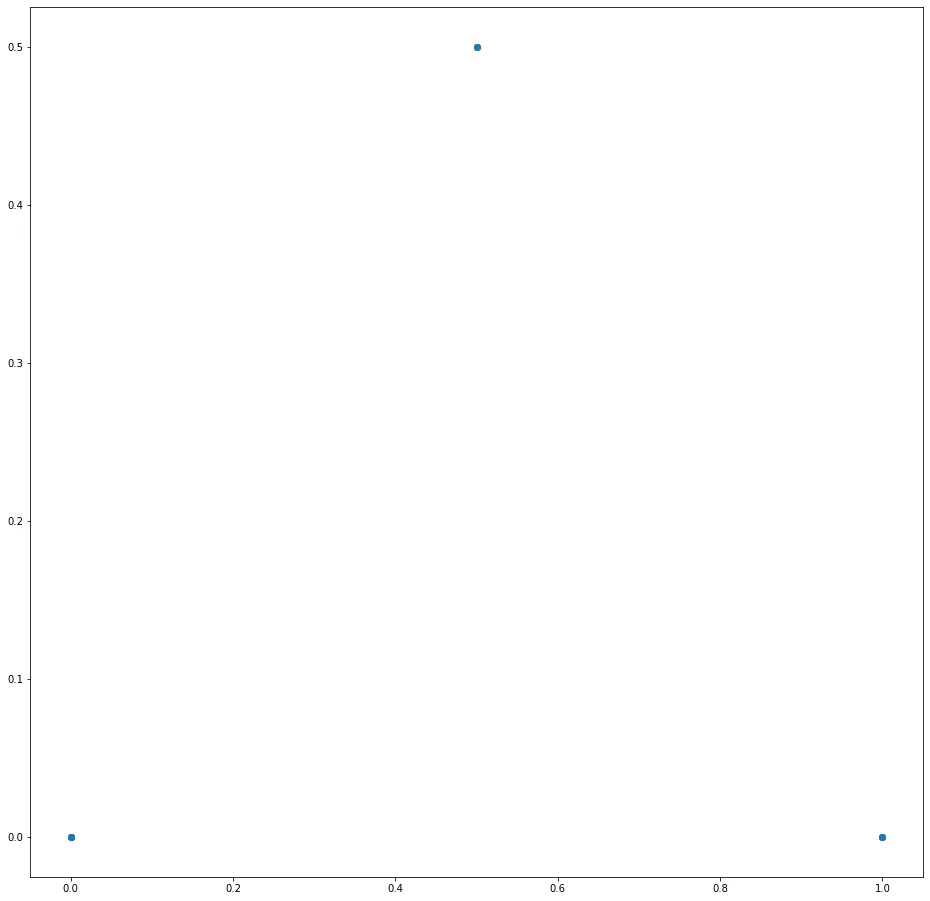07 Aug 2023

Posted on:

03 Aug 2023

0

# Resolved:Program not working. Please check!

def trans1(x,y):
x1=0.5*x
y1=0.5*y
return x1,y1

def trans2(x,y):
x1=0.5*x+0.5
y1=0.5*y+0.5
return x1,y1

def trans3(x,y):
x1=0.5*x + 1
y1=0.5*y
return x1,y1

def sierpinski_triangle(x=0,y=0,n=10):
'''Sierpinski Triangle'''

transformation=[trans1,trans2,trans3]
a1,b1=[0.0],[0.0]
for i in range(n):
trans=choice(transformation)
a,b=trans(x,y)
a1.append(a)
b1.append(b)
plt.rc('figure',figsize=(16,16))
plt.plot(a1,b1,'o')

sierpinski_triangle()

The output is coming same for all range from 10-1000.Instructor
Posted on:

07 Aug 2023

0

Hey Arkajit,

Thank you for reaching out!

Note the definition of your `sierpinski_triangle()` function

``def sierpinski_triangle(x=0,y=0,n=1000)``

as well as the following line of code

``a,b=trans(x,y)``

Calling `trans()` with parameters `x` and `y` results in evaluating the function at `x=0` and `y=0` each time. You will get three different outcomes depending on the choice of transformation function:

- The function `trans1(x=0, y=0)` would always return `(0, 0)`.

- The function `trans2(x=0, y=0)` would always return `(0.5, 0.5)`.

- The function `trans3(x=0, y=0)` would always return `(1, 0)`.

This is the reason why you plot only these three points in your graph.

To remedy this, change the following line of code

``a,b=trans(x,y)``

``a,b=trans(a,b)``

In this way, you will always update the latest values of the variables `a` and `b`. Of course, you should also specify their initial values. You would therefore need to add the following line of code before the `for`-loop:

``a, b = 0.0, 0.0``

Having done that, the parameters x and y become redundant and can be removed from the definition of the `sierpinski_triangle()` function.

The final look of the function is the following:

``````def sierpinski_triangle(n=1000):
'''Sierpinski Triangle'''

transformation=[trans1,trans2,trans3]
a1,b1=[0.0],[0.0]
a, b = 0.0, 0.0
for i in range(n):
trans=choice(transformation)
a,b=trans(a,b)
a1.append(a)
b1.append(b)
plt.rc('figure',figsize=(16,16))
plt.plot(a1,b1,'o')``````

Hope this helped! Good luck on your Python journey!

Kind regards,

365 Hristina